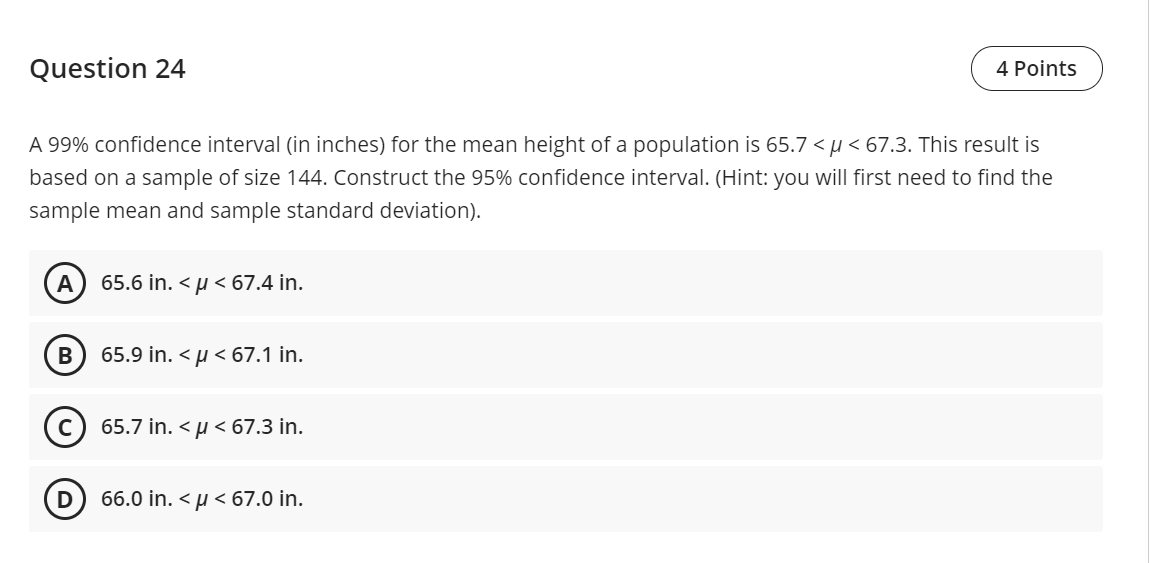💬 👋 We’re always here. Join our Discord to connect with other students 24/7, any time, night or day.Join Here!

KT

Karla N T.

Intro Stats / AP Statistics

1 week, 5 days ago

# A 99% confidence interval (in inches) for the mean height of a population is 65.7 < ? < 67.3. This result is based on a sample of size 144. Construct the 95% confidence interval. (Hint: you will first need to find the sample mean and sample standard deviation).Michigan State University

A 99% confidence interval (in inches) for the mean height of a population is 65.7 < ? < 67.3. This result is based on a sample of size 144. Construct the 95% confidence interval. (Hint: you will first need to find the sample mean and sample standard deviation).

### Discussion

You must be signed in to discuss.

### Video Transcript

So you have a 99% confidence interval and I see, you may have asked this question yesterday and I didn't see that you had a multiple choice answer. And so in any case We have that is the interval for 99% and you want to find a 95% confidence interval. So we know that the mean of this sample is going to be 65.7, 67.3 divided by two or the mean of that, The middle of that number, and that comes out to be 66.5. And we also know that the difference between uh this is 66.5 plus or minus some and a Z value times the sample standard deviation over the square root of and and we know that we're going to use approximately a Z due to the fact that this sample size is large enough to use as the So we have to figure out what this component, is we know that for a 99% confidence, interval. We know that the two points 576 is the Z value that we'd use. We don't know the sample standard deviation And we know that the sample size is 144 and that margin of air is the difference between the mean and the 67.3 or the 65.7- is 66.5. And so we find out that that difference is .6 Uh .8, I'm Sorry. So if we subtract these two numbers, we get .8 or if we subtract these two numbers, we're going to end up getting .8 and so we can find what the sample standard deviation is by taking uh The .8 and multiplying it by 12 And then dividing by the 2.576. And we find that that sample standard deviation comes up to be 3.7267. So there's our sample standard deviation and here is our X bar. So now we want to find the 95% confidence interval, so we're going to take that 66.5 Plus or minus and the z star value for 95%, is that 1.960 And times the sample standard deviation, which is that 3.7267 divided by the square root of the sample size. And your answer is multi is rounded off to the nearest 10th. So we just need to know what that margin of air is to the nearest 10th And we find out that this value right here comes out to be plus or -16. So when we subtract away .6 we go down to 65.9 And when we add on the .6 we go up to 67.1. So that Is your 95% confidence interval rounded off to the nearest temp. And that I believe is your alternative beMichigan State University
##### Top Intro Stats / AP Statistics Educators##### Catherine R.

Missouri State University##### Michael J.

Idaho State UniversityLectures

Join Bootcamp